# GMAT Math : Calculating the angle of a sector

## Example Questions

### Example Question #1 : Calculating The Angle Of A Sector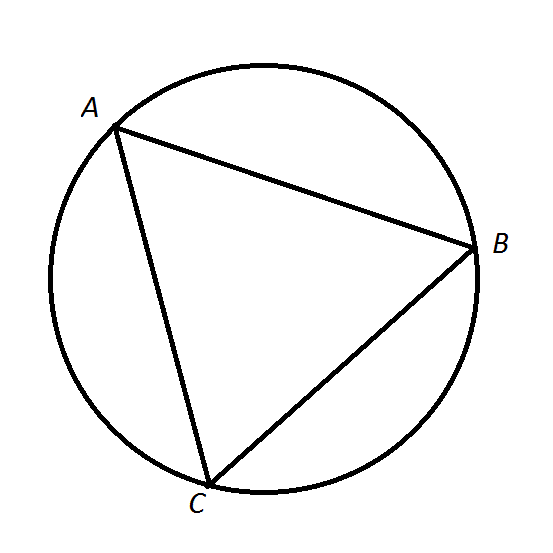Note: Figure NOT drawn to scale.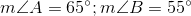.

Order the degree measures of the arcs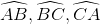from least to greatest.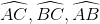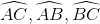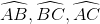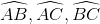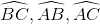Explanation: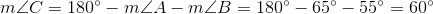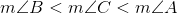, so, by the Multiplication Property of Inequality,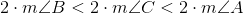.

The degree measure of an arc is twice that of the inscribed angle that intercepts it, so the above can be rewritten as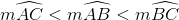.

### Example Question #2 : Calculating The Angle Of A Sector

In the figure shown below, line segment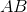passes through the center of the circle and has a length of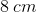. Points, andare on the circle. Sector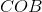covers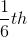of the total area of the circle. Answer the following questions regarding this shape.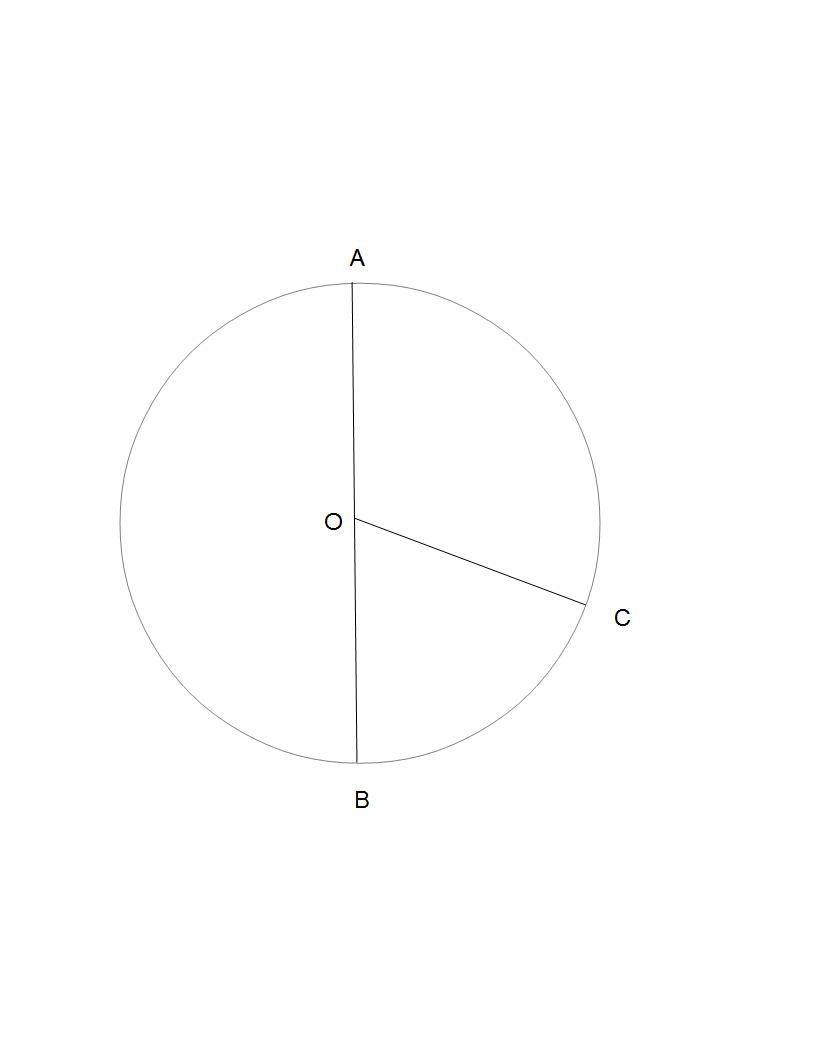Find the value of central angle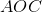.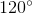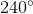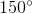Explanation:

Here we need to recall the total degree measure of a circle. A circle always has exactly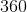degrees.

Knowing this, we need to utilize two other clues to find the degree measure of.

1) Angle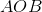measuresdegrees, because it is made up of line segment, which is a straight line.

2) Angle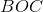can be found by using the following equation. Because we are given the fractional value of its area, we can construct a ratio to solve for angle: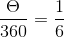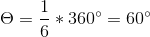So, to find angle, we just need to subtract our other values from: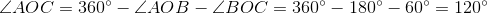So,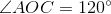.

### Example Question #3 : Calculating The Angle Of A Sector

The radius of Circle A is equal to the perimeter of Square B. A sector of Circle A has the same area as Square B. Which of the following is the degree measure of this sector?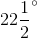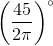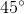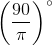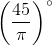Explanation:

Call the length of a side of Square B. Its perimeter is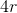, which is the radius of Circle A.

The area of the circle is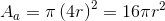; that of the square is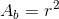. Therefore, a sector of the circle with area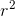will be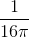of the circle, which is a sector of measure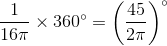### Example Question #4 : Calculating The Angle Of A Sector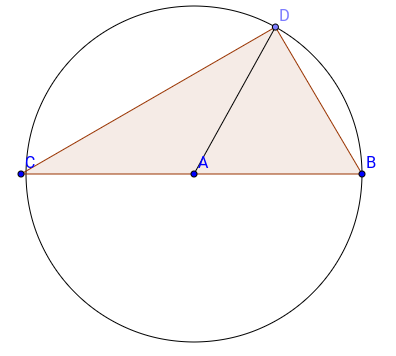Angle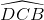is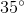. What is angle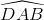?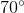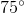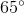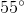Explanation:

This is the kind of question we can't get right if we don't know the trick. In a circle, the size of an angle at the center of the circle, formed by two segments intercepting an arc, is twice the size of the angle formed by two lines intercepting the same arc, provided one of these lines is the diameter of the circle. in other words,is twice.

Thus,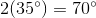### Example Question #5 : Calculating The Angle Of A Sector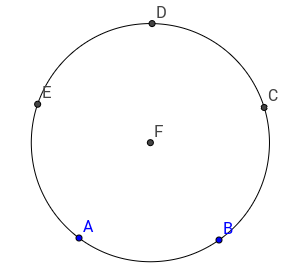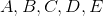areevenly spaced points on the circle. What is angle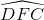?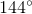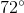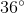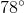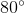Explanation:

We can see that the points devide the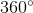of the circle in 5 equal portions.

The final answer is given simply by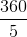which is, this is the angle of a slice of a pizza cut in 5 parts if you will!

### Example Question #6 : Calculating The Angle Of A Sector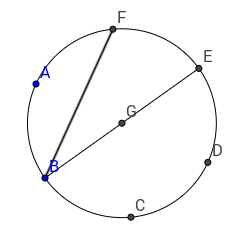Thepointsandare evenly spaced on the circle of center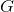. What is the size of angle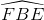?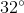As we have seen previously, the 6 points divide theof the circle in 6 portion of same angle. Each portion form an angle of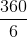or 60 degrees. As we also have previously seen, the angle formed by the lines intercepting an arc is twice more at the center of the circle than at the intersection of the lines intercepting the same arc with the circle, provided one of these lines is the diameter. In other words,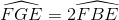. Since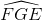is 60 degrees, than,must be 30 degrees, this is our final answer.